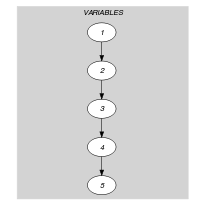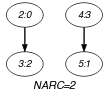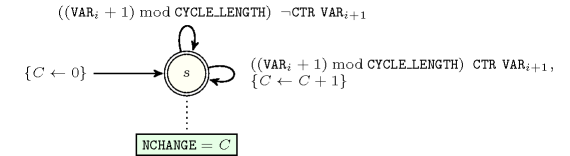## 5.107. cyclic_change

Origin

Derived from $\mathrm{𝚌𝚑𝚊𝚗𝚐𝚎}$.

Constraint

$\mathrm{𝚌𝚢𝚌𝚕𝚒𝚌}_\mathrm{𝚌𝚑𝚊𝚗𝚐𝚎}\left(\mathrm{𝙽𝙲𝙷𝙰𝙽𝙶𝙴},\mathrm{𝙲𝚈𝙲𝙻𝙴}_\mathrm{𝙻𝙴𝙽𝙶𝚃𝙷},\mathrm{𝚅𝙰𝚁𝙸𝙰𝙱𝙻𝙴𝚂},\mathrm{𝙲𝚃𝚁}\right)$

Arguments
 $\mathrm{𝙽𝙲𝙷𝙰𝙽𝙶𝙴}$ $\mathrm{𝚍𝚟𝚊𝚛}$ $\mathrm{𝙲𝚈𝙲𝙻𝙴}_\mathrm{𝙻𝙴𝙽𝙶𝚃𝙷}$ $\mathrm{𝚒𝚗𝚝}$ $\mathrm{𝚅𝙰𝚁𝙸𝙰𝙱𝙻𝙴𝚂}$ $\mathrm{𝚌𝚘𝚕𝚕𝚎𝚌𝚝𝚒𝚘𝚗}\left(\mathrm{𝚟𝚊𝚛}-\mathrm{𝚍𝚟𝚊𝚛}\right)$ $\mathrm{𝙲𝚃𝚁}$ $\mathrm{𝚊𝚝𝚘𝚖}$
Restrictions
 $\mathrm{𝙽𝙲𝙷𝙰𝙽𝙶𝙴}\ge 0$ $\mathrm{𝙽𝙲𝙷𝙰𝙽𝙶𝙴}<|\mathrm{𝚅𝙰𝚁𝙸𝙰𝙱𝙻𝙴𝚂}|$ $\mathrm{𝙲𝚈𝙲𝙻𝙴}_\mathrm{𝙻𝙴𝙽𝙶𝚃𝙷}>0$ $\mathrm{𝚛𝚎𝚚𝚞𝚒𝚛𝚎𝚍}$$\left(\mathrm{𝚅𝙰𝚁𝙸𝙰𝙱𝙻𝙴𝚂},\mathrm{𝚟𝚊𝚛}\right)$ $\mathrm{𝚅𝙰𝚁𝙸𝙰𝙱𝙻𝙴𝚂}.\mathrm{𝚟𝚊𝚛}\ge 0$ $\mathrm{𝚅𝙰𝚁𝙸𝙰𝙱𝙻𝙴𝚂}.\mathrm{𝚟𝚊𝚛}<\mathrm{𝙲𝚈𝙲𝙻𝙴}_\mathrm{𝙻𝙴𝙽𝙶𝚃𝙷}$ $\mathrm{𝙲𝚃𝚁}\in \left[=,\ne ,<,\ge ,>,\le \right]$
Purpose

$\mathrm{𝙽𝙲𝙷𝙰𝙽𝙶𝙴}$ is the number of times that constraint $\left(\left(X+1\right)\mathrm{mod}\mathrm{𝙲𝚈𝙲𝙻𝙴}_\mathrm{𝙻𝙴𝙽𝙶𝚃𝙷}\right)\mathrm{𝙲𝚃𝚁}Y$ holds; $X$ and $Y$ correspond to consecutive variables of the collection $\mathrm{𝚅𝙰𝚁𝙸𝙰𝙱𝙻𝙴𝚂}$.

Example
$\left(2,4,〈3,0,2,3,1〉,\ne \right)$

Since $\mathrm{𝙲𝚃𝚁}$ is set to $\ne$ and since $\mathrm{𝙲𝚈𝙲𝙻𝙴}_\mathrm{𝙻𝙴𝙽𝙶𝚃𝙷}$ is set to 4, a change between two consecutive items $X$ and $Y$ of the $\mathrm{𝚅𝙰𝚁𝙸𝙰𝙱𝙻𝙴𝚂}$ collection corresponds to the fact that the condition $\left(\left(X+1\right)\mathrm{mod}4\right)\ne Y$ holds. Consequently, the $\mathrm{𝚌𝚢𝚌𝚕𝚒𝚌}_\mathrm{𝚌𝚑𝚊𝚗𝚐𝚎}$ constraint holds since we have the two following changes (i.e., $\mathrm{𝙽𝙲𝙷𝙰𝙽𝙶𝙴}=2$) within $〈3,0,2,3,1〉$:

• A first change between the consecutive values 0 and 2,

• A second change between the consecutive values 3 and 1.

However, the sequence $30$ does not correspond to a change since $\left(3+1\right)\mathrm{mod}4$ is equal to 0.

Typical
 $\mathrm{𝙽𝙲𝙷𝙰𝙽𝙶𝙴}>0$ $|\mathrm{𝚅𝙰𝚁𝙸𝙰𝙱𝙻𝙴𝚂}|>1$ $\mathrm{𝚛𝚊𝚗𝚐𝚎}$$\left(\mathrm{𝚅𝙰𝚁𝙸𝙰𝙱𝙻𝙴𝚂}.\mathrm{𝚟𝚊𝚛}\right)>1$ $\mathrm{𝙲𝚃𝚁}\in \left[\ne \right]$
Symmetry

Items of $\mathrm{𝚅𝙰𝚁𝙸𝙰𝙱𝙻𝙴𝚂}$ can be shifted.

Arg. properties

Functional dependency: $\mathrm{𝙽𝙲𝙷𝙰𝙽𝙶𝙴}$ determined by $\mathrm{𝙲𝚈𝙲𝙻𝙴}_\mathrm{𝙻𝙴𝙽𝙶𝚃𝙷}$, $\mathrm{𝚅𝙰𝚁𝙸𝙰𝙱𝙻𝙴𝚂}$ and $\mathrm{𝙲𝚃𝚁}$.

Usage

This constraint may be used for personnel cyclic timetabling problems where each person has to work according to cycles. In this context each variable of the $\mathrm{𝚅𝙰𝚁𝙸𝙰𝙱𝙻𝙴𝚂}$ collection corresponds to the type of work a person performs on a specific day. Because of some perturbation (e.g., illness, unavailability, variation of the workload) it is in practice not reasonable to ask for perfect cyclic solutions. One alternative is to use the $\mathrm{𝚌𝚢𝚌𝚕𝚒𝚌}_\mathrm{𝚌𝚑𝚊𝚗𝚐𝚎}$ constraint and to ask for solutions where one tries to minimise the number of cycle breaks (i.e., the variable $\mathrm{𝙽𝙲𝙷𝙰𝙽𝙶𝙴}$).

Keywords
Arc input(s)

$\mathrm{𝚅𝙰𝚁𝙸𝙰𝙱𝙻𝙴𝚂}$

Arc generator
$\mathrm{𝑃𝐴𝑇𝐻}$$↦\mathrm{𝚌𝚘𝚕𝚕𝚎𝚌𝚝𝚒𝚘𝚗}\left(\mathrm{𝚟𝚊𝚛𝚒𝚊𝚋𝚕𝚎𝚜}\mathtt{1},\mathrm{𝚟𝚊𝚛𝚒𝚊𝚋𝚕𝚎𝚜}\mathtt{2}\right)$

Arc arity
Arc constraint(s)
$\left(\mathrm{𝚟𝚊𝚛𝚒𝚊𝚋𝚕𝚎𝚜}\mathtt{1}.\mathrm{𝚟𝚊𝚛}+1\right)\mathrm{mod}\mathrm{𝙲𝚈𝙲𝙻𝙴}_\mathrm{𝙻𝙴𝙽𝙶𝚃𝙷}\mathrm{𝙲𝚃𝚁}\mathrm{𝚟𝚊𝚛𝚒𝚊𝚋𝚕𝚎𝚜}\mathtt{2}.\mathrm{𝚟𝚊𝚛}$
Graph property(ies)
$\mathrm{𝐍𝐀𝐑𝐂}$$=\mathrm{𝙽𝙲𝙷𝙰𝙽𝙶𝙴}$

Graph class
 $•$$\mathrm{𝙰𝙲𝚈𝙲𝙻𝙸𝙲}$ $•$$\mathrm{𝙱𝙸𝙿𝙰𝚁𝚃𝙸𝚃𝙴}$ $•$$\mathrm{𝙽𝙾}_\mathrm{𝙻𝙾𝙾𝙿}$

Graph model

Parts (A) and (B) of Figure 5.107.1 respectively show the initial and final graph associated with the Example slot. Since we use the $\mathrm{𝐍𝐀𝐑𝐂}$ graph property, the arcs of the final graph are stressed in bold.

##### Figure 5.107.1. Initial and final graph of the $\mathrm{𝚌𝚢𝚌𝚕𝚒𝚌}_\mathrm{𝚌𝚑𝚊𝚗𝚐𝚎}$ constraint(a) (b)
Automaton

Figure 5.107.2 depicts the automaton associated with the $\mathrm{𝚌𝚢𝚌𝚕𝚒𝚌}_\mathrm{𝚌𝚑𝚊𝚗𝚐𝚎}$ constraint. To each pair of consecutive variables $\left({\mathrm{𝚅𝙰𝚁}}_{i},{\mathrm{𝚅𝙰𝚁}}_{i+1}\right)$ of the collection $\mathrm{𝚅𝙰𝚁𝙸𝙰𝙱𝙻𝙴𝚂}$ corresponds a 0-1 signature variable ${S}_{i}$. The following signature constraint links ${\mathrm{𝚅𝙰𝚁}}_{i}$, ${\mathrm{𝚅𝙰𝚁}}_{i+1}$ and ${S}_{i}$: $\left(\left({\mathrm{𝚅𝙰𝚁}}_{i}+1\right)\mathrm{mod}\mathrm{𝙲𝚈𝙲𝙻𝙴}_\mathrm{𝙻𝙴𝙽𝙶𝚃𝙷}\right)\mathrm{𝙲𝚃𝚁}{\mathrm{𝚅𝙰𝚁}}_{i+1}⇔{S}_{i}$.

##### Figure 5.107.2. Automaton of the $\mathrm{𝚌𝚢𝚌𝚕𝚒𝚌}_\mathrm{𝚌𝚑𝚊𝚗𝚐𝚎}$ constraint##### Figure 5.107.3. Hypergraph of the reformulation corresponding to the automaton of the $\mathrm{𝚌𝚢𝚌𝚕𝚒𝚌}_\mathrm{𝚌𝚑𝚊𝚗𝚐𝚎}$ constraint